Courses

Test: Alligation or Mixture- 2

10 Questions MCQ Test Quantitative Aptitude (Quant) | Test: Alligation or Mixture- 2

Description
Attempt Test: Alligation or Mixture- 2 | 10 questions in 20 minutes | Mock test for Quant preparation | Free important questions MCQ to study Quantitative Aptitude (Quant) for Quant Exam | Download free PDF with solutions
QUESTION: 1

Solution:
QUESTION: 2

Solution:
QUESTION: 3

A vessel is filled with liquid, 3 parts of which are water and 5 parts of syrup. How much of the mixture must be drawn off and replaced with water so that the mixture may be half water and half syrup?

Solution:

Let initially vessel have 8 l of liquid and x lof this liquid be replaced with water then quantity of water in new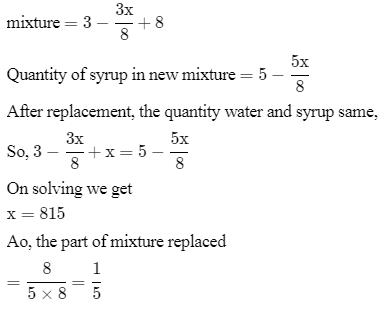QUESTION: 4

A container contains a mixture of two liquids P and Q in the ratio 7 : 5. When 9 litres of mixture are drawn off and the container is filled with Q, the ratio of P and Q becomes 7 : 9. How many litres of liquid P was contained in the container initially?

Solution: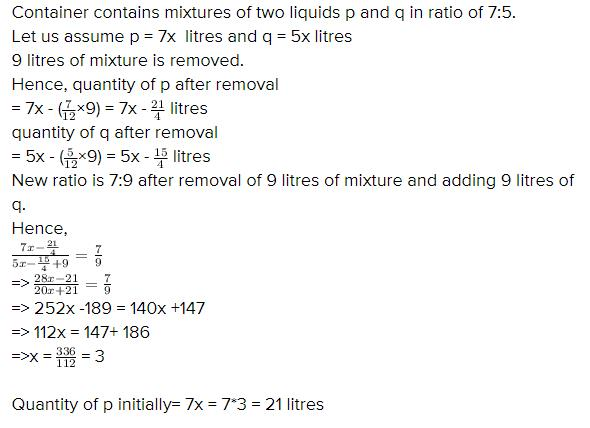QUESTION: 5

In what ratio must a grocer mix two varieties of pulses costing Rs.15 and Rs. 20 per kg respectively to obtain a mixture worth Rs.16.50 per Kg?

Solution: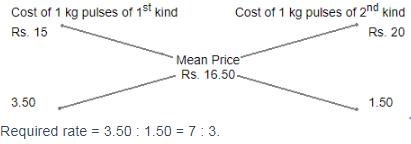QUESTION: 6

In what ratio must tea worth Rs. 60 per kg be mixed with tea worth Rs. 65 a Kg such that by selling the mixture at Rs. 68.20 a Kg ,there can be a gain 10%?

Solution:

100/110 × 68.20 = 62
Let the amount of tea worth rs 60 be x and the amount of tea worth rs 65 be y
The total amount is : x + y
60x + 65y = 62(x + y)
60x + 65y = 62x + 62y
65y - 62y = 62x - 60x
3y = 2x
3/2 = x / y
3 : 2

QUESTION: 7

A merchant has 1000 kg of sugar part of which he sells at 8% profit and the rest at 18% profit. He gains 14% on the whole. The Quantity sold at 18% profit is

Solution:

Let the sugar sold at 8% gain = x
Therefore, Sugar sold at 18% gain = (1000 - x)
Let CP of sugar = Rs.y per kg
Total CP = Rs.100y
Therefore,
(108/100 * xy) + 118/100(1000-x)y
114/100*1000y
= 108xy + 118000y - 118xy = 114000y
10x = 4000
x = 400
Quantity sold at 18% profit
= 1000 - 400 = 600 kg

QUESTION: 8

A dishonest milkman sells his milk at cost price but he mixes it with water and thereby gains 25%. What is the percentage of water in the mixture?

Solution:

Let C.P. of 1 litre milk be Re. 1
Then, S.P. of 1 litre of mixture = Re. 1, Gain = 25%.
C.P. of 1 litre mixture = Re.(100/125 * 1) = 4/5
By the rule of alligation, we have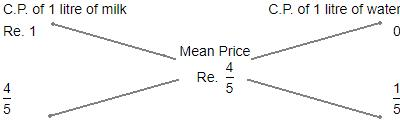Ratio of milk to water = 4/5 : 1/5
=> 4:5
Hence, percentage of water in the mixture = 1/5 x 100%
= 20%

QUESTION: 9

In what ratio must water be mixed with milk costing Rs.12 per litre in order to get a mixture worth of Rs.8 per litre?

Solution:

Let's assume cost of water is 0,
And the amount of water in mixture be x litres, and amount of milk in mixture be y litres,
So as per question the mixture would be sold a 8 Rs/litre
=> Cost of water per litre times amount of water in mixture of 1 litre + Cost of milk per litre times amount of milk in mixture of 1 litre must be equal to required cost of 1 litre mixture times 1 litre(total mixture quantity)
Mathematically, 0*x + 12*y = 8*1
=> 12 y = 8
=> y=2/3 litre
Now since total mixture is 1 litre,
So x = 1-y
x = 1-(2/3) = 1/3 litre
So ratio of water to milk in mixture, to get cost of required mixture to 1 litre = x/y = (1/3)/(2/3)
=> Ratio of water to milk in mixture is 1:2

QUESTION: 10

In what ratio must water be mixed with milk to gain 16 2/3 % on selling the mixture at cost price?

Solution:

Let C.P. of 1 litre milk be Rs. 1
S.P. of 1 litre of mixture = Rs. 1, Gain =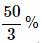∴ C.P. of 1 litre of mixture =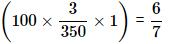BY the rule of alligation, we have: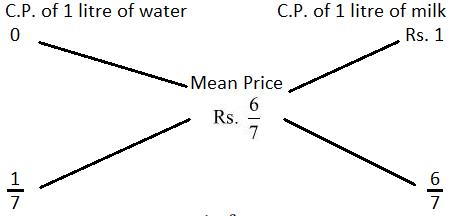∴ Ration of water and milk =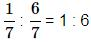Use Code STAYHOME200 and get INR 200 additional OFF Use Coupon Code

Track your progress, build streaks, highlight & save important lessons and more!

Similar ContentRelated tests